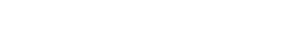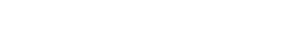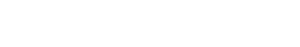# Excel Formula Final Question #4 from Modeloff 2013 Released with Explanation (4 of 4)

The long awaited final question from Modeloff 2013 is here! Sorry for keeping you all waiting so long, KeyCuts has been going through some really awesome developments and we have not had a lot of time to blog. Our goal is to increase our blogging to once a week so expect awesome stories around Excel, analysis, and career insights in the future! What have we been working on you ask? Stay tuned, in the next couple of weeks we will release details about a partnership with an online education platform to help everyone around the world learn Excel through the latest trend in MOOCs (Massive Open Online Course).

Challenge number 4 was the most difficult challenge at Modeloff 2013. So hard, in fact, none of the contestants were able to solve the challenge in the allotted time. The teams were furiously experimenting with different formulas but as the clock started ticking down, a few simply gave up with exasperated looks on their faces; fingers resting on the keyboard instead of tirelessly typing new formulas to solve the challenge. If you can solve this challenge, you are in the top .1% of modelers out there, and you should definitely pre-register for Modeloff 2014! If you want to take a look at the first 3 challenges from 2013 Modeloff series, here are the questions along with the explanations:

As always, thanks to Dan Mayoh, the creator of all these challenges for providing us with the answers. Dan’s consulting practice is online now, so go check out Fintega Pty Ltd for more info. Now, onto the challenge!

## The Final Excel Formula Challenge

Take a look at the first post in this series to familiarize yourself with the rules of the challenge. No VBA, Defined Names, or any other Excel black magic. Your formula must be entered into the grid of blue cells below:There is also a 5 X 2 table of dates and duration periods that will help you with writing the formula:Don’t forget! There is also a table of answers in the Excel file (download) that will turn green if the formula you entered into the blue answer cells is correct:## The Objective

Write a formula that counts the number of cumulative flags to date that have been “set off” for each period end date (above the blue answer cells) relative to the first flag date. For instance, if the period end date is December 31st, 2014, and the first flag date is December 31st, 2013, and the duration of the flag is 1 year, the answer for the number of flags set off is 2. The answer is 2 because December 31st, 2013 is in the past (relative to the period end date), and a 1 year has passed since December 31st, 2013, so the flag was set off again on December 31st, 2014.

As another example, consider the period end date to be June 30th, 2015. The first flag date is June 30th, 2014, but the duration is 0.5 years. This means every 6 months the flag gets set off. The answer in this scenario is 3 since the flag was set off on June 30th, 2014, December 29th, 2014, and finally June 30th, 2014. In cases where the period end date is BEFORE the first flag date, the answer would simply be 0 since the flag would never be set off in the first place.

Ready to get your ass kicked? Or become a legendary financial modeler? Download the Excel file here that the contestants were given and try to solve the challenge yourself! The goal is to find the formula that satisfies the conditions of the challenge using the least number of characters possible in the formula.

## Initial Formula Attempts

Knowing that none of the contestants were able to solve this challenge, I was easily discouraged from trying to spend too much time banging my head to figure out the solution. I did take a few stabs at writing the formula as I knew it would involve dividing the difference between the period end date and the first flag date by 365. For instance, in cell O19 I started with this:

`=(O\$19-\$E19)/365`

You need to add the “\$” to the row in O19 and to the column in E19 so that when you copy the formula across the blue cells, the reference to the period end date and first flag dates to not move around in the formula and stay fixed. I also knew that the formula would probably involve dividing the number of days by the duration cell, so the formula would look something like this in O19:

`=(O\$19-\$E19)/365/\$F19`

This is pretty much where I got stuck as there are a few things you need to account for in the formula which remained unanswered to me:

• How do you account for the edge cases where the flag needs to increment by 1 given that subtracting the period end date from the flag date and then dividing by 365 still results in 1?
• How do you ensure that the answer is 0 for the period end dates that are before the flag date (columns H-J)?
• Piggy backing off of that last question, do we use the MIN() function somewhere to make sure we get the right answer for when the period end date is before the flag date?

There are still many unknowns, and the main problem I had was handling the edge cases (as is the case with most of the challenges in this series). Just finding a solution that works is difficult enough, forget trying to find the formula with the least number of characters.How it feels to be stuck in Excel.

## The Solution

The final solution contains 34 characters and only uses the INT() and MIN() functions:

=-INT(MIN(\$E19-H\$17-9,1)/365/\$F19)

This formula is entered into cell H19 (not array-entered) and copied across and down to show all the flags in the blue answer cells:## The Explanation

I was surprised myself that the final formula only utilized the INT() and MIN() functions, but through the clever use of these two functions we are able to see the edge cases handled elegantly.

Let’s take a look at each segment of the final formula to see how this formula is able to handle all the edge cases. Let’s take the formula in cell Q19 as an example:

`=-INT(MIN(\$E19-Q\$17-9,1)/365/\$F19)`

\$E19 equals December 31st, 2013 and Q\$17 equals the period end date, June 30th, 2014. Let’s forget the “-9” for now and see what happens when we just evaluate =MIN(\$E19-Q\$17,1). The result is -546, which is essentially the difference in days between December 31st, 2013 and June 30th, 2015. The reason why MIN() evaluates to -546 is because this function takes in the two arguments, -546 and 1 and returns the lesser of the two arguments. Now let’s divide by 365 and we see the answer is is -1, or -1.50 if you show two decimal places.

This is starting to make sense now. The difference between December 31st, 2013 and June 30th, 2015 is indeed 1.50 years. If we divide the -1.50 by the duration, we will still get an answer of -1.5 since the flag duration is 1 year. Now let’s add in the INT() function and see how it affects the formula:

`=INT(MIN(\$E19-Q\$17-9,1)/365/\$F19)`

The result is -2, so we are definitely on the right track here. Why does =INT(-1.5) evaluate to -2? You usually use the INT() function to get the integer of a decimal number. For instance, the INT() function would return 5 for the number 5.25. However, for negative numbers, the INT() function returns the first negative number that is less than or equal to the expression. You would think that =INT(-1.5) would result in -1 (the integer of -1.5) but the closest integer to -1.5 that is less than -1.5 is -2. Even if the expression is -1.001, =INT(-1.001) still evaluates to -2.

The final solution has a “-” in front of it so this would turn the -2 to a 2 which is the correct value for cell Q19.

## The Purpose of the “-9” in the Final Solution

This leads us to the question about the “-9” which we omitted in our explanation above. It looks like this formula yields the correct answer for us:

`=-INT(MIN(\$E19-Q\$17,1)/365/\$F19)`

If you copy this formula across the rest of the blue answer cells, however, you’ll start to notice some errors in the table of answers. The reason is because the -9 helps us with edge cases when the period end date is the same as the flag date or one or two years ahead of the flag date. For instance, let’s look at the formula in cell Q20 without the -9:

=-INT(MIN(\$E20-Q\$17,1)/365/\$F20)

The result is 2 but this is incorrect since the actual answer is 3. The flag date is June 30th, 2014 and the period end date is June 30th, 2015, exactly one year ahead of the flag date. The duration is only 0.50 years in this case. This means that the flag has been set off 3 times: once on June 30th, 2014, again on December 31st, 2014, and one final time on June 30th, 2015. The issue with the formula above is that \$E20-Q\$17 results in -365. When we take -365 and divide it by 365 and then by the duration, 0.50, we will get the -2 answer which we currently see. What we need is a number to decrease the -365 so that when we divide by 365 the answer is not exactly -1, but rather a little less than -1. Then, when we incorporate the INT() function with the given expression, the result will will be the lesser negative number, or -3 in this case.

Let’s add in the -9 to the formula and see what happens:

=MIN(\$E20-Q\$17-9,1)/365/\$F20)

The result is -2.05, which is slightly less than -2 but makes a world of difference in terms of what the INT() function evaluates the expression to. The lesser number of -2.05 is -3, and when we multiply this number with the minus sign at the beginning of the formula, we will get the 3 that we need in cell Q20. There is no specific reason why the solution uses a “-9,” we could also use “-1” and the answers in the blue cells will be correct. The important concept here is to decrease the negative result of the the flag date minus the period end date by a little bit for the INT() function to evaluate the expression correctly.

## Conclusion of the Modeloff 2013 Excel Challenges

This challenge shows how a formula may appear to work, but once you analyze the edge cases, you’ll begin to see how the formula breaks down and you need to incorporate other functions or elements to make the formula work. The INT() function wouldn’t even be incorporate into the formula if it wasn’t for the “-9” since the purpose of the INT() is to find the lesser integer from the expression. If we assumed the formula worked without the “-9,” the resulting formula would’ve been a lot shorter and wouldn’t have caused so much anguish among the Modeloff contestants.

Through all these Modeloff challenges, the one common theme I see is to observe the details about how data that is fed into the formulas. What may seem like a straightforward formula may become very complicated as you deal with the edge cases, as we saw with the final challenge. Hopefully these challenges helped you learn a little more about building advanced formulas in Excel, and some of the real-life issues analysts, consultants, and modelers face on their job.